PolymathPlus

# PolymathPlus

Math-Solver for students, scientists, and engineers
Enter a problem in plain text   Press the Solve button   Get a numeric solution

 Linear Equations Nonlinear Equations Differential Equations Curve Fitting Regression

PolymathPlus is free and will always remain free for students and professionals.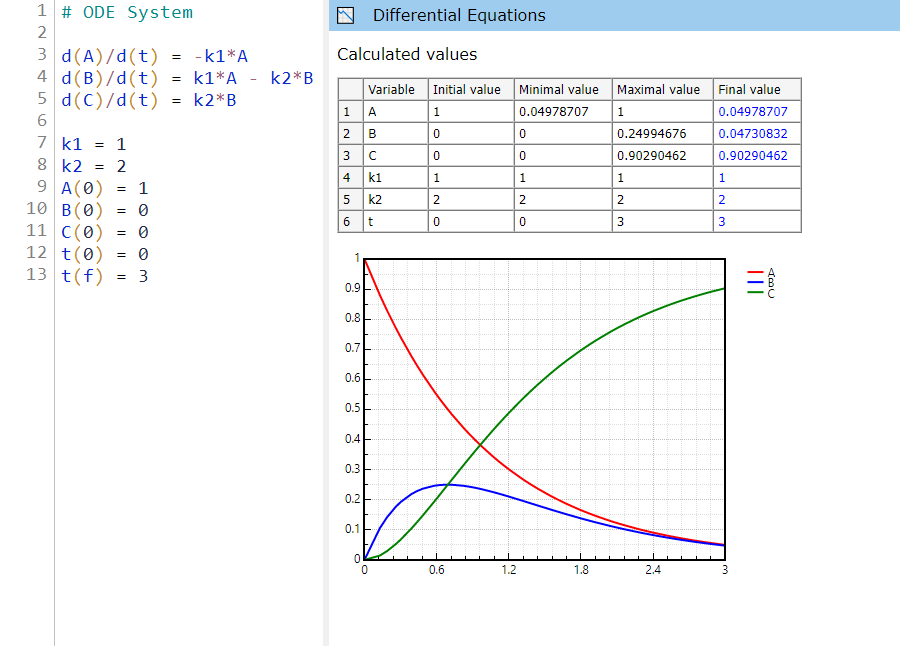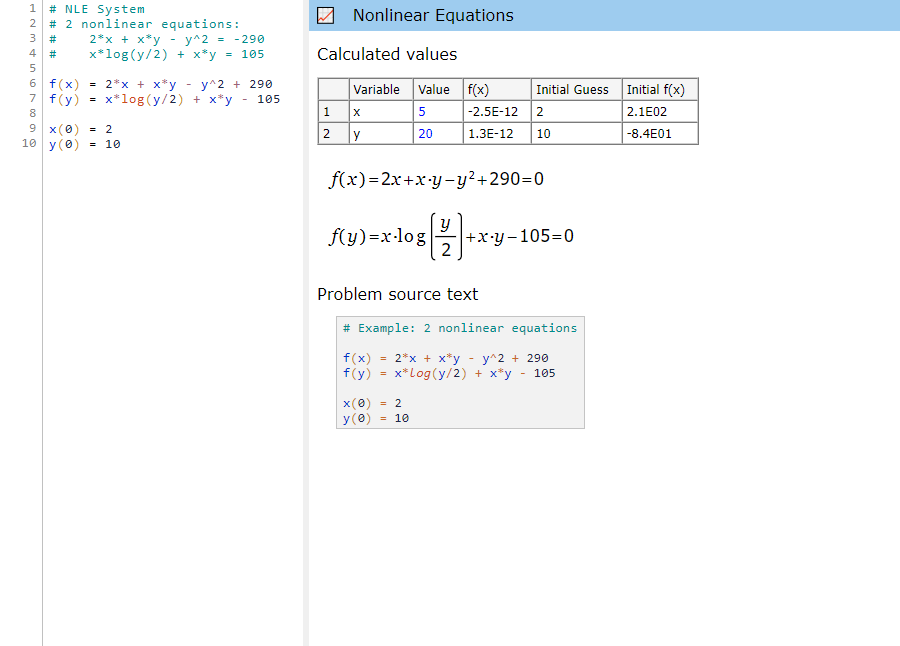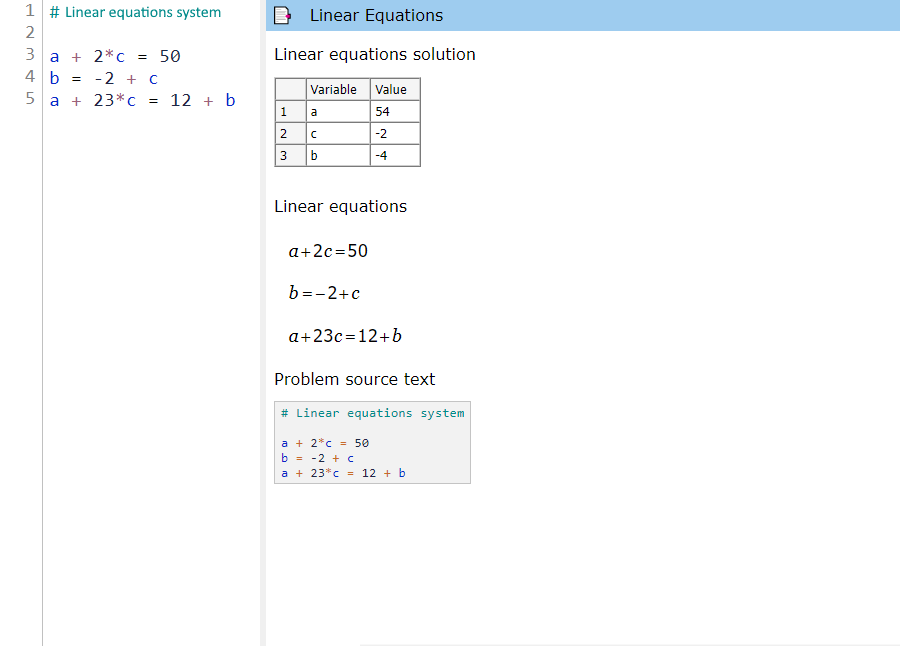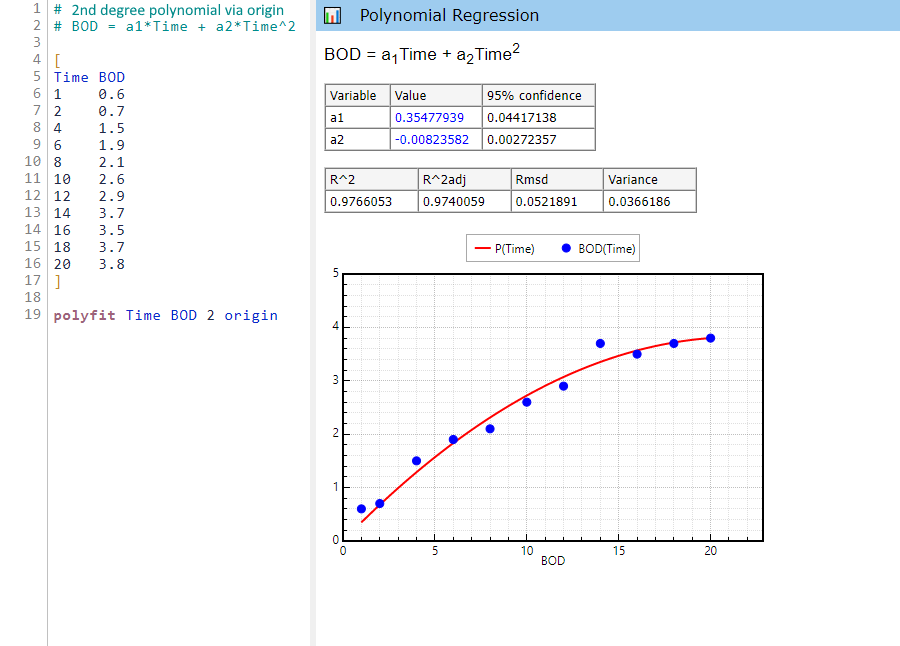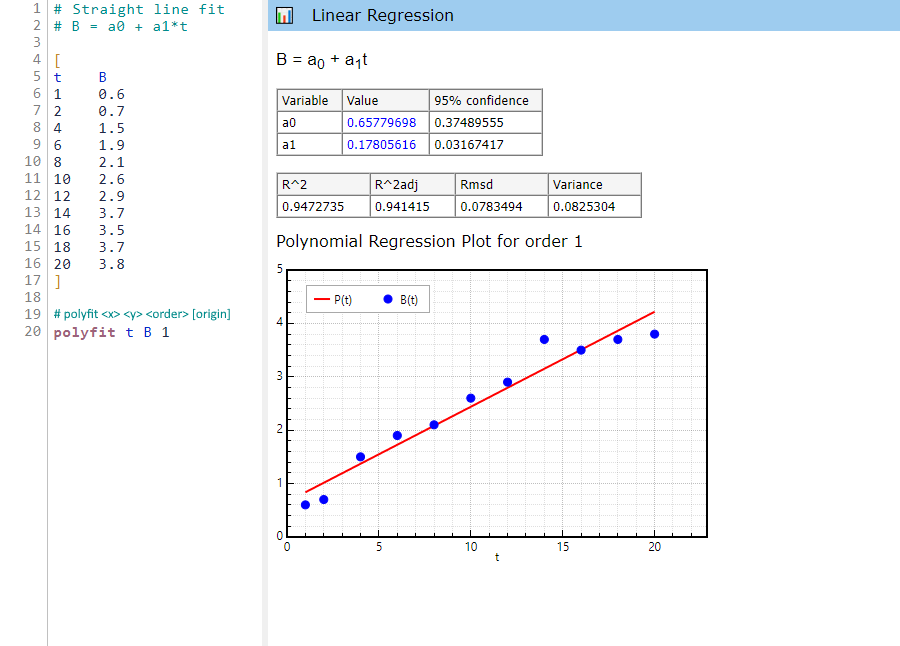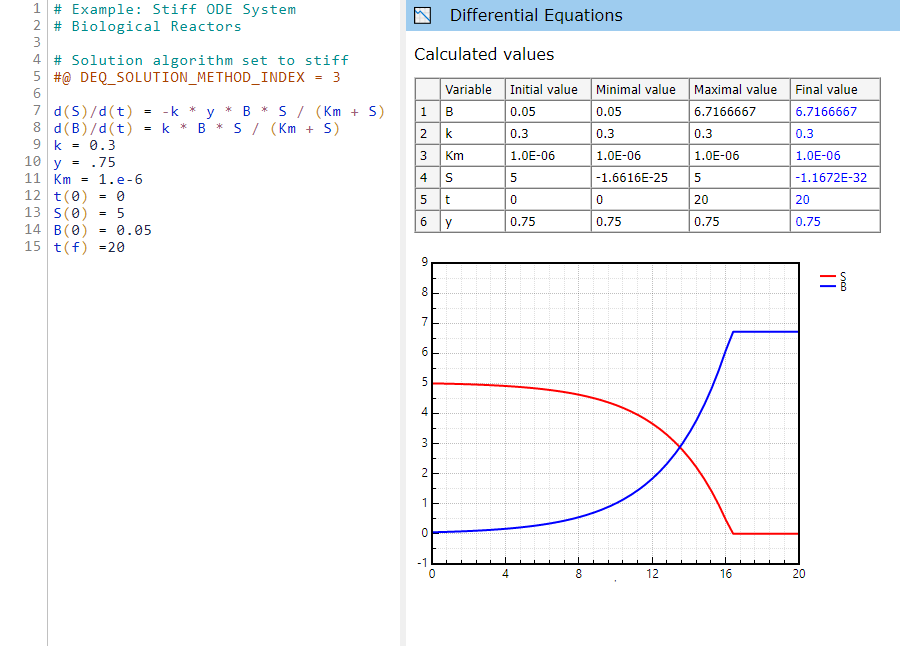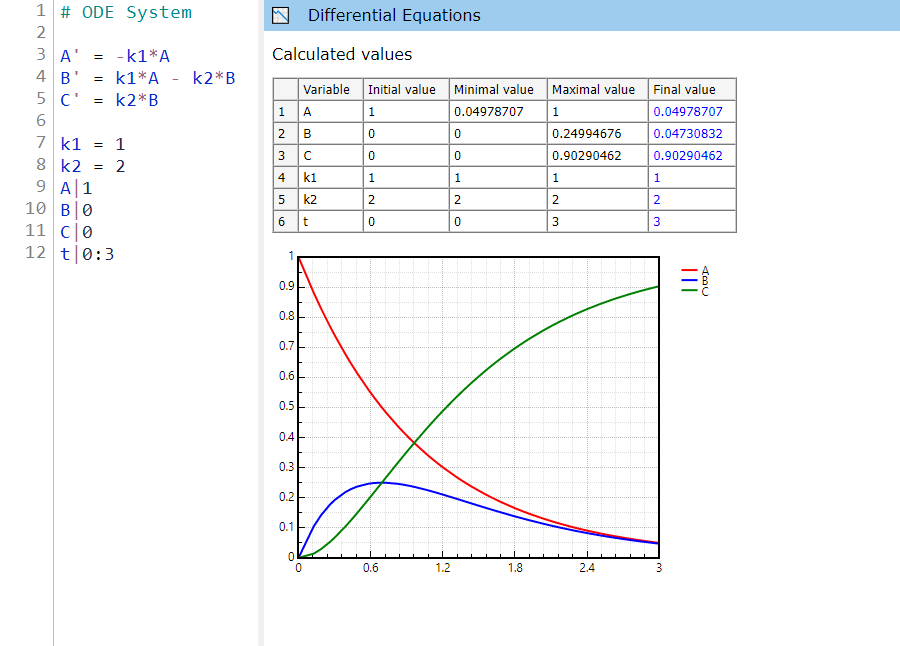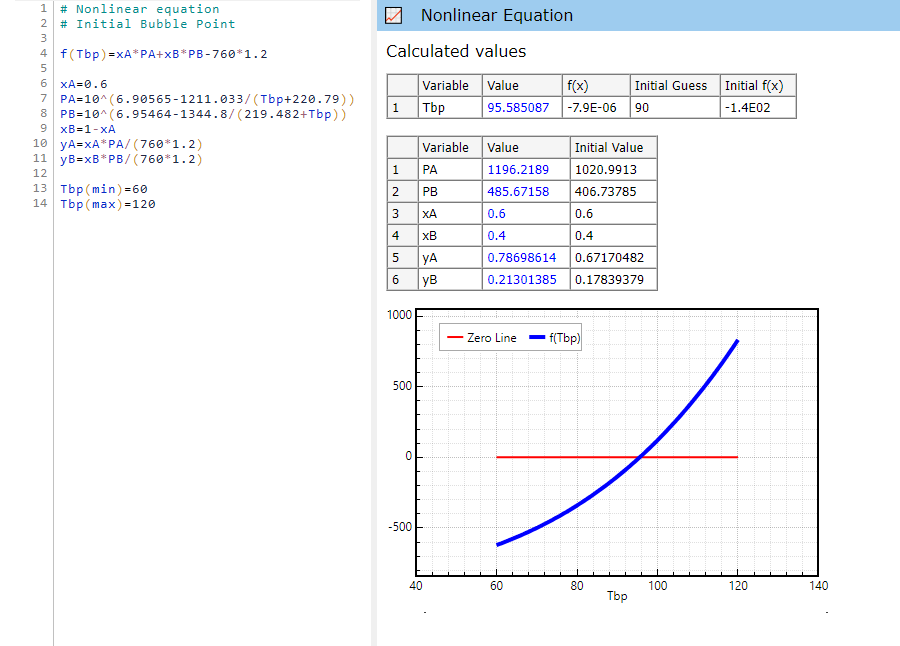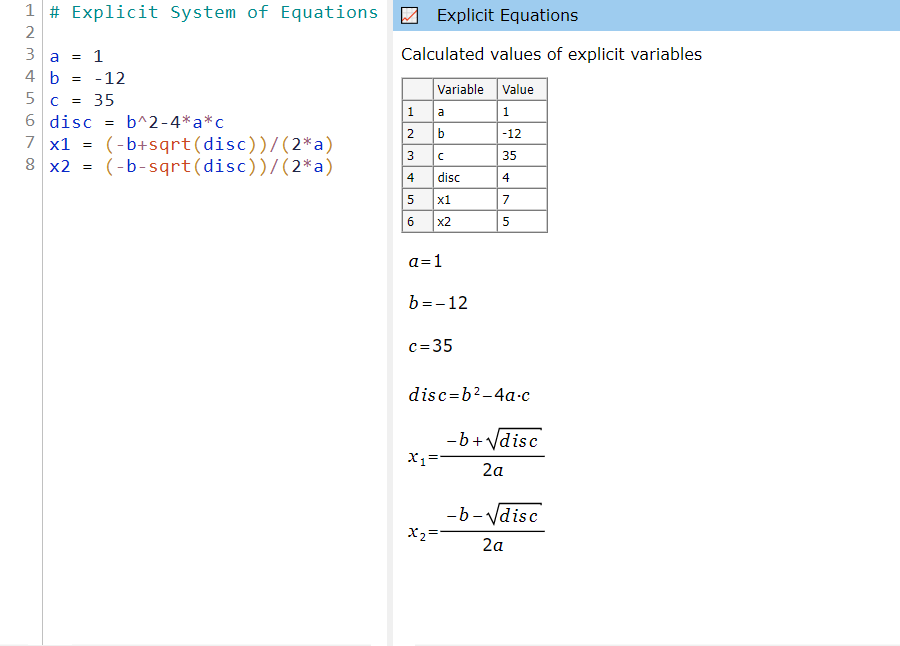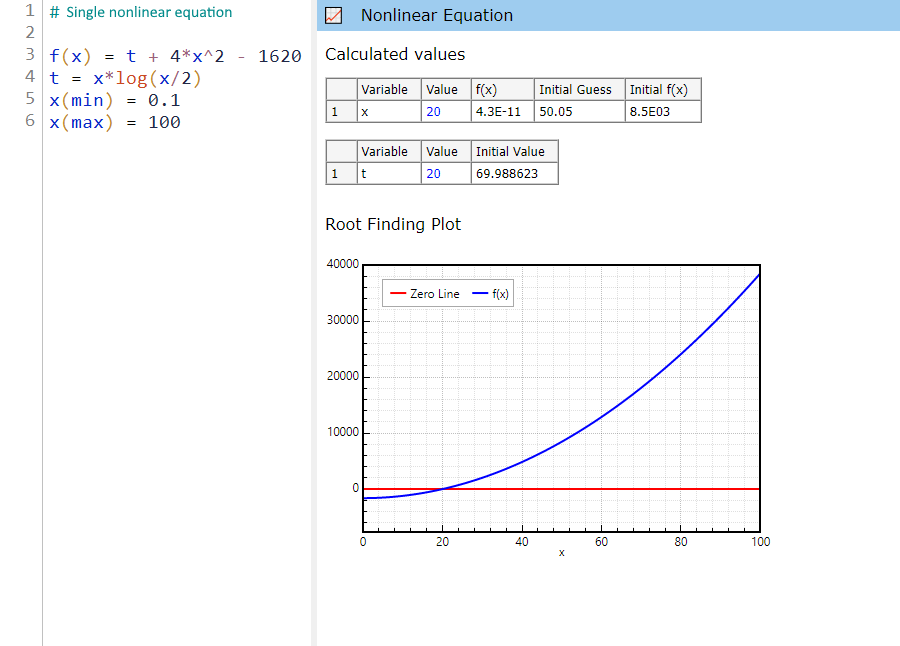The PolymathPlus solver is available as a web application. A link to the solver becomes visible on the left menubar after login. Please note that the Windows desktop application is no longer supported.

Our internal algorithms have been extensively used over the years by many engineering faculties in universities across the world. Students and professionals will appreciate the ease of use and the comprehensive solution reports of our application.

## Supported Web BrowsersChrome 85Safari 14Edge 85Firefox 79Opera 71

** Internet-Explorer is not supported.

# Modules

### Linear Equations

PolymathPlus can solve linear equations of any size. The number of equations should be equal to the number of unknown linear variables. Here's an example of 3 linear equations to be solved simultaneously:Below are the equations entered into the PolymathPlus problem input text box (note that the # sign indicates user remarks and is being ignored by the program):

# linear equations a + 2*c = 50 b = -2 + c a + 23*c = 12 + b

### Nonlinear Equations

When solving simultaneous nonlinear equations, the equations have to be written as root expressions (expressions that are equal to zero in the solution). In addition, an initial guess should be provided for each nonlinear variable.
Below is a sample problem and data entry for solving 2 nonlinear equations:When entering the problem into PolymathPlus, we should enter the root expressions, each associated to a nonlinear variable function. We should also provide an initial estimate for each nonlinear variable. In the example below, we have 2 nonlinear variables x and y, hence 2 root functions, and we provide an initial guess of 1 for both x, and y.

f(x) = x^2 + y - 12 f(y) = 2*x + log(y+2) - 5 x(0)=1 y(0)=1

Note that we may also define any number of auxiliary expressions, to simplify long expressions, in this example we define `a` as an auxiliary expression:

f(x) = x^2 + y - 12 f(y) = 2*x + a a = log(y+2) - 5 x(0)=1 y(0)=1

In case we only have one nonlinear equation to solve (with any number of auxiliary expressions), we need to provide a min/max estimates for the nonlinear variable. For example, below is a PolymathPlus program for solving one nonlinear equation where we need to evaluate `V`:

f(V) = (P+a/(V^2))*(V-b)-R*T P=56 R=0.08206 T=450 Tc=405.5 Pc=111.3 Pr=P/Pc a=27*(R^2*Tc^2/Pc)/64 b=R*Tc/(8*Pc) Z=P*V/(R*T) V(min)=0.4 V(max)=1

### Differential Equations

Entering and solving ordinary differential equations with PolymatPlus is simple.
Consider this set of equations to be solved:Given the initial conditions x0=1, y0=4, at t0=0, and having the integration finish at tf=1,
the text below should be entered into PolymathPlus:

x' = x + 4*t^2 y' = sqrt(y) - x/(5-y) x|1 y|4 t|0:1

The following alternative compatible syntax can also be used for the same problem:

d(x)/d(t) = x + 4*t^2 d(y)/d(t) = sqrt(y) - x/(5-y) x(0)=1 y(0)=4 t(0)=0 t(f)=1

The solution will be displayed in a tabular format and will also include the integration chart: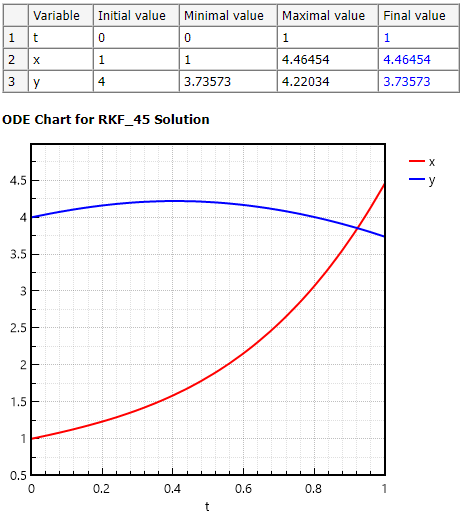### Curve Fitting

PolymathPlus supports curve fitting for polynomial, multi-linear, and nonlinear regression. The report evaluates the model variables, generates a regression chart, residual plots, and provides statistics on the accuracy of the model.

Here is an example of the data entry required for solving a linear regression model for a given set of data points.
We solve a polynomial regression model with the order of 1 (hence linear regression) which best fits the linear equation matching the given data points.

`polyfit <x> <y> <order> [origin]`

# Linear regression example # Straight line fit to BOD data # BOD = a0 + a1*Time [ t BOD 1 0.6 2 0.7 4 1.5 6 1.9 8 2.1 10 2.6 12 2.9 14 3.7 16 3.5 18 3.7 20 3.8 ] Time = t + 10 polyfit Time BOD 1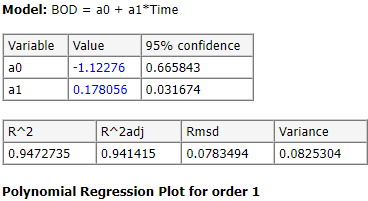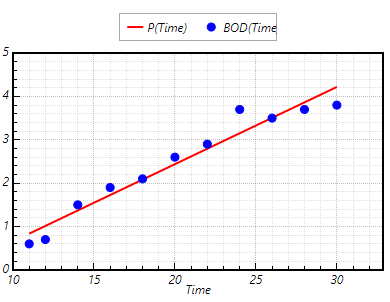Below is an example of a data entry for solving nonlinear regression model for a given set of data points. The model variables to be found are `a` and `b`, for which we should also provide an initial guess.

# Example: Nonlinear Regression [ x y g 0.5 1.255 1.2 0.387 1.25 1.3 0.24 1.189 1.4 0.136 1.124 2.0 0.04 0.783 2.1 0.011 0.402 2.2 ] # Nonlinear regression model nlinfit y = a * x / (b + x) # Initial guess of the # regression model variables a,b m(a)= 2 m(b)= 1

More examples can be found in this Help file.

# Register

User registration is needed to access the online solver which becomes visible after login.

Please don't use the form above to register the application on a public or cloud computers, site IT administrators should get a site registration license instead.

This section is relevant for academic/other organizations looking to use PolymathPlus on their public lab or cloud computers.

To register a new site, first contact us with the following details: (1) Site name (2) IT contact email (3) Alternate email (4) Email extension/s for the organization. Then, we will provide an access key for the site. The instructions to install and register PolymathPlus over multiple computers will be available via the access form below.